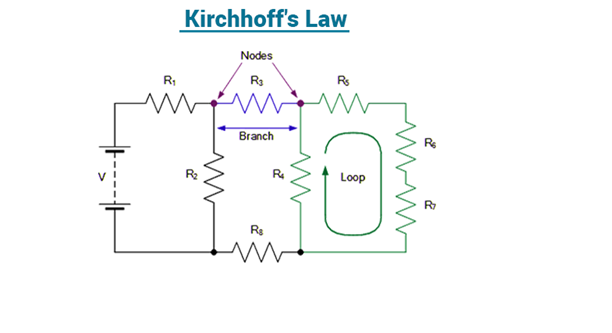# Kirchoff’s Current and voltage laws## Introduction

The current in various branches of large network can not be found out by ordinary methods. By applications kirchoff’s laws, complicated networks can be solved. Gustav Robert Kirchoff( 1824 – 1887) a German physicist, published the first systematic description of the laws of circuit analysis. These laws are known as Kirchoff’s current law (KCL) and Kirchoff’s voltage law ( KVL). His contribution forms the basis of all circuit analysis problems

1) Current law : or point law It States that , in any net work of wires carrying currents the algebraic sum of the currents meeting at Junction ( or point) is zero. It is also called as point lawThis can also be defined as the total currents flowing towards a Junction is equal to that total currents flowing away from the junction.

2) Mesh law or voltage law : In any closed electric circuit, the sum of potential drops ( I.R) is equal to the sum of the impressed e.m.f.s.## Application of Kirchoff’s laws to the wheat stone bridge :

Wheat stone bridge is used to measure the unknown resistance in a given network. It consists of four arms. If the current in the galvanometer is zero it is called a balanced. Circuit, then the products of the resistance of opposite
arms are equal. Suppose the value of current through the galvanometer is not zero then the kirchoffs laws are applied to find the currents and values of unknown resistance then it is called an ‘unbalanced’ bridge circuit.